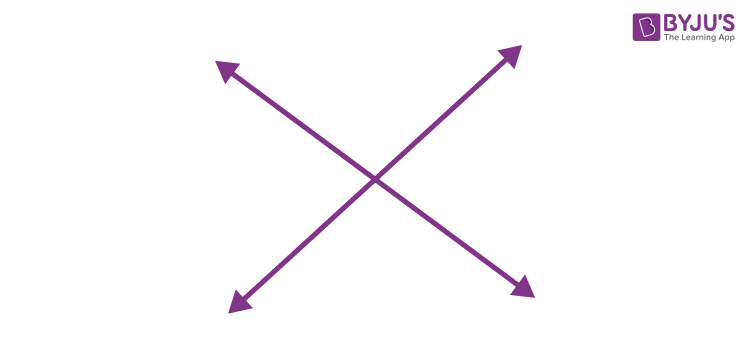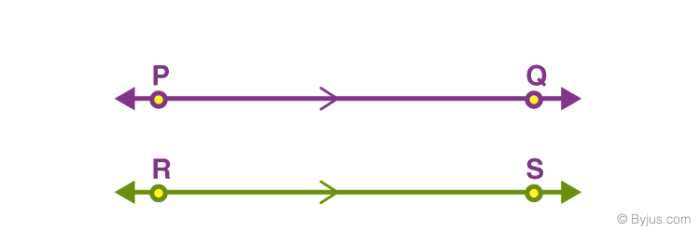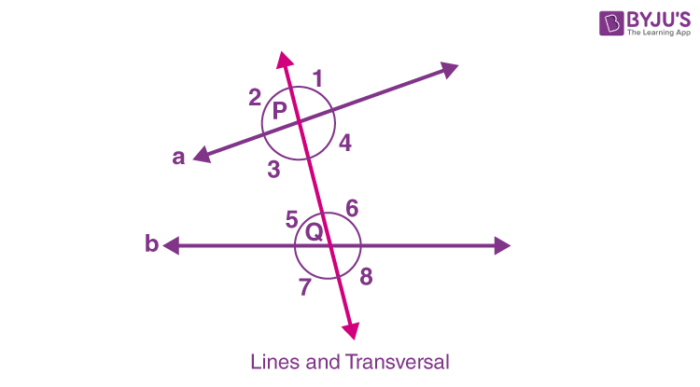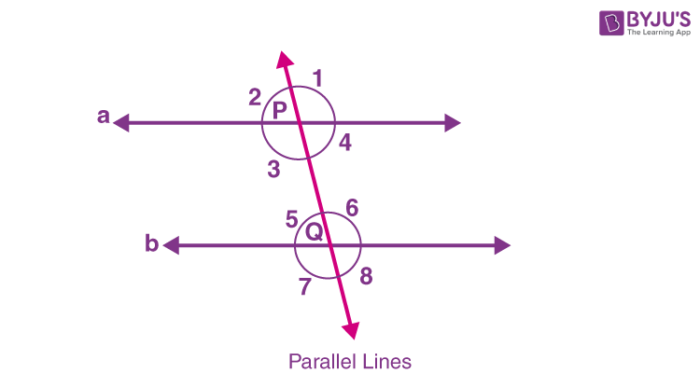# Pairs of Lines

In Geometry, pairs of lines are the lines that are connected or related to each other. In Class 7 chapter lines and angles, we will come across three different pairs of lines, such as:

## Intersecting Lines

The pairs of lines that intersect at a common point are called intersecting lines. The point where they intersect is known as the point of intersection.

We can also say, the pair of lines segments or rays are said to be intersecting, when they intersect at a single point. For example, the letter X has two line segments that intersect at a single point.## Parallel Lines

The pairs of lines that do not intersect or meet at any point are called parallel lines. These lines lie on the same plane but do not intersect.

The line segments or rays that lie on the same plane but do not intersect are also parallel.In the above example, the two line segments PQ and RS are parallel to each other.

In real life also we can see the examples of parallel lines such as edges of the ruler are parallel, railway tracks are parallel and extend indefinitely.

## Transversals

A line that intersects two or more lines at a distinct point is known as a transversal line or just a transversal.

As we already know by intersecting lines, when they meet at a point, angles are formed. In the same way, when a transverse line intersects two or more lines, at each intersection point, different angles are formed.

For example, a transversal intersects two lines as shown in the figure.In the above given figure, transversal intersects the lines a and b at point P and Q, respectively. Thus, the pairs of angles formed at P and Q are:

• Interior angles: ∠3, ∠4, ∠5, ∠6
• Exterior angles: ∠1, ∠2, ∠7, ∠8
• Pairs of Corresponding angles: ∠1 and ∠6, ∠2 and ∠5, ∠3 and ∠7, ∠4 and ∠8
• Pairs of Alternate interior angles: ∠3 and ∠6, ∠4 and ∠5
• Pairs of Alternate exterior angles: ∠1 and ∠7, ∠2 and ∠8
• Pairs of interior angles on the same side of the transversal: ∠3 and ∠5, ∠4 and ∠6

But the above pairs of angles are formed for two non-parallel lines here. Thus, we cannot say if any of the angles here are of the same measure. Hence, we will take the pairs of lines that are parallel to each other and intersected by a transversal.

### Transversal of Parallel Lines

Now, we can discuss the angles formed by the pair of lines when a transversal intersects the parallel lines.In the above figure, we can see that a transversal intersects the parallel lines a and b at point P and Q. Thus, the angles so formed will have the below properties here.

 Each pair of corresponding angles are equal ∠1 = ∠6, ∠2 = ∠5, ∠3 = ∠7, ∠4 = ∠8 Each pair of alternate interior angles are equal ∠3 = ∠6, ∠4 = ∠5 Each pairs of alternate exterior angles are equal ∠1 = ∠7, ∠2 = ∠8 Each pairs of interior angles on the same side of the transversal are supplementary ∠3 + ∠5 = 180°, ∠4 + ∠6 = 180°

## Frequently Asked Questions on Pairs of Lines

### What are pairs of lines in geometry?

In geometry, pairs of lines are those lines that are related to each other.

### What are the types of lines in pairs?

There are basically three pairs of lines. They are intersecting lines, parallel lines and transversal lines.

### What are pairs of parallel lines?

The lines that are parallel to each other and do not intersect at any point are called parallel lines.

### How many pairs of intersecting lines are there?

When two or more lines meet at a single point, they are called intersecting lines. Thus, there have to be at least two lines to form intersecting lines in a plane.

### What angles are formed when two lines intersect?

When two lines intersect at a common point, then vertically opposite angles are formed.